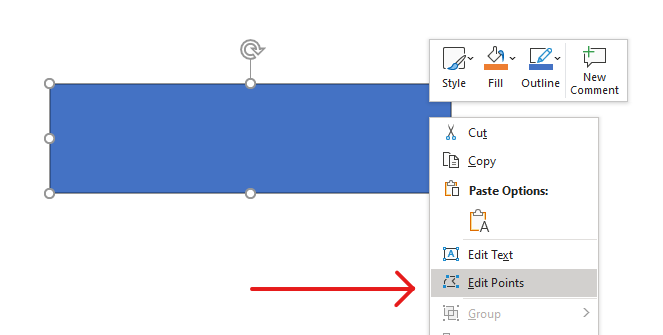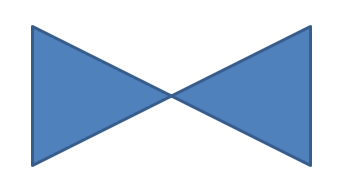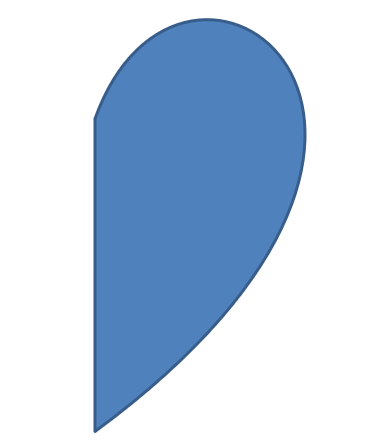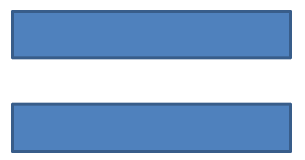Contents
[ ]

# Change a Shape Using Edit Points

Consider a square. In PowerPoint, using edit points, you can

• move the square’s corner in or out
• specify the curvature for a corner or point
• add new points to the square
• manipulate points on the square, etc.

Essentially, you can perform the described tasks on any shape. Using edit points, you get to change a shape or create a new shape from an existing shape.

## Shape Editing TipsBefore you start editing PowerPoint shapes through edit points, you might want to consider these points about shapes:

• A shape (or its path) can either be closed or open.
• When a shape is closed, it lacks a start or end point. When a shape is open, it has a beginning and end.
• All shapes consist of at least 2 anchor points linked to each other by lines
• A line is either straight or curved. Anchor points determine the nature of the line.
• Anchor points exist as corner points, straight points, or smooth points:
• A corner point is a point where 2 straight lines join at an angle.
• A smooth point is a point where 2 handles exist in a straight line and the line’s segments join in a smooth curve. In this case, all handles are separated from the anchor point by an equal distance.
• A straight point is a point where 2 handles exist in a straight line and that line’s line segments joins in a smooth curve. In this case, the handles don’t have to be separated from the anchor point by an equal distance.
• By moving or editing anchor points (which changes the angle of lines), you can change the way a shape looks.

To edit PowerPoint shapes through edit points, Aspose.Slides provides the GeometryPath class and IGeometryPath interface.

## Simple Editing Operations

This C# code shows you how to

Add a line to the end of a path

``````void LineTo(PointF point);
void LineTo(float x, float y);
``````

Add a line to a specified position on a path:

``````void LineTo(PointF point, uint index);
void LineTo(float x, float y, uint index);
``````

Add a cubic Bezier curve at the end of a path:

``````void CubicBezierTo(PointF point1, PointF point2, PointF point3);
void CubicBezierTo(float x1, float y1, float x2, float y2, float x3, float y3);
``````

Add a cubic Bezier curve to the specified position on a path:

``````void CubicBezierTo(PointF point1, PointF point2, PointF point3, uint index);
void CubicBezierTo(float x1, float y1, float x2, float y2, float x3, float y3, uint index);
``````

``````void QuadraticBezierTo(PointF point1, PointF point2);
void QuadraticBezierTo(float x1, float y1, float x2, float y2);
``````

``````void QuadraticBezierTo(PointF point1, PointF point2, uint index);
void QuadraticBezierTo(float x1, float y1, float x2, float y2, uint index);
``````

Append a given arc to a path:

``````void ArcTo(float width, float heigth, float startAngle, float sweepAngle);
``````

Close the current figure of a path:

``````void CloseFigure();
``````

Set the position for the next point:

``````void MoveTo(PointF point);
void MoveTo(float x, float y);
``````

Remove the path segment at a given index:

``````void RemoveAt(int index);
``````

## Add Custom Points to Shape

1. Create an instance of the GeometryShape class and set the ShapeType.Rectangle type.
2. Get an instance of the GeometryPath class from the shape.
3. Add a new point between the two top points on the path.
4. Add a new point between the two bottom points on the path.
5. Apply the path to the shape.

This C# code shows you how to add custom points to a shape:

``````using (Presentation pres = new Presentation())
{
GeometryShape shape = pres.Slides.Shapes.AddAutoShape(ShapeType.Rectangle, 100, 100, 200, 100) as GeometryShape;
IGeometryPath geometryPath = shape.GetGeometryPaths();

geometryPath.LineTo(100, 50, 1);
geometryPath.LineTo(100, 50, 4);
shape.SetGeometryPath(geometryPath);
}
``````## Remove Points From Shape

1. Create an instance of GeometryShape class and set the ShapeType.Heart type.
2. Get an instance of the GeometryPath class from the shape.
3. Remove the segment for the path.
4. Apply the path to the shape.

This C# code shows you how to remove points from a shape:

``````using (Presentation pres = new Presentation())
{
GeometryShape shape = pres.Slides.Shapes.AddAutoShape(ShapeType.Heart, 100, 100, 300, 300) as GeometryShape;

IGeometryPath path = shape.GetGeometryPaths();
path.RemoveAt(2);
shape.SetGeometryPath(path);
}
``````## Create Custom Shape

1. Calculate the points for the shape.
2. Create an instance of the GeometryPath class.
3. Fill the path with the points.
4. Create an instance of the GeometryShape class.
5. Apply the path to the shape.

This C# shows you how to create a custom shape:

``````List<PointF> points = new List<PointF>();

float R = 100, r = 50;
int step = 72;

for (int angle = -90; angle < 270; angle += step)
{
double radians = angle * (Math.PI / 180f);
double x = R * Math.Cos(radians);
double y = R * Math.Sin(radians);
points.Add(new PointF((float)x + R, (float)y + R));

radians = Math.PI * (angle + step / 2) / 180.0;
points.Add(new PointF((float)x + R, (float)y + R));
}

GeometryPath starPath = new GeometryPath();
starPath.MoveTo(points);

for (int i = 1; i < points.Count; i++)
{
starPath.LineTo(points[i]);
}

starPath.CloseFigure();

using (Presentation pres = new Presentation())
{
GeometryShape shape = pres.Slides.Shapes.AddAutoShape(ShapeType.Rectangle, 100, 100, R * 2, R * 2) as GeometryShape;

shape.SetGeometryPath(starPath);
}
``````## Create Composite Custom Shape

1. Create an instance of the GeometryShape class.
2. Create a first instance of the GeometryPath class.
3. Create a second instance of the GeometryPath class.
4. Apply the paths to the shape.

This C# code shows you to create a composite custom shape:

``````using (Presentation pres = new Presentation())
{
GeometryShape shape = pres.Slides.Shapes.AddAutoShape(ShapeType.Rectangle, 100, 100, 200, 100) as GeometryShape;

GeometryPath geometryPath0 = new GeometryPath();
geometryPath0.MoveTo(0, 0);
geometryPath0.LineTo(shape.Width, 0);
geometryPath0.LineTo(shape.Width, shape.Height/3);
geometryPath0.LineTo(0, shape.Height / 3);
geometryPath0.CloseFigure();

GeometryPath geometryPath1 = new GeometryPath();
geometryPath1.MoveTo(0, shape.Height/3 * 2);
geometryPath1.LineTo(shape.Width, shape.Height / 3 * 2);
geometryPath1.LineTo(shape.Width, shape.Height);
geometryPath1.LineTo(0, shape.Height);
geometryPath1.CloseFigure();

shape.SetGeometryPaths(new GeometryPath[] { geometryPath0, geometryPath1});
}
``````## Create Custom Shape With Curved Corners

This C# code shows you how to create a custom shape with curved corners (inwards);

``````var shapeX = 20f;
var shapeY = 20f;
var shapeWidth = 300f;
var shapeHeight = 200f;

var leftTopSize = 50f;
var rightTopSize = 20f;
var rightBottomSize = 40f;
var leftBottomSize = 10f;

using (var presentation = new Presentation())
{
ShapeType.Custom, shapeX, shapeY, shapeWidth, shapeHeight);

var geometryPath = new GeometryPath();

var point1 = new PointF(leftTopSize, 0);
var point2 = new PointF(shapeWidth - rightTopSize, 0);
var point3 = new PointF(shapeWidth, shapeHeight - rightBottomSize);
var point4 = new PointF(leftBottomSize, shapeHeight);
var point5 = new PointF(0, leftTopSize);

geometryPath.MoveTo(point1);
geometryPath.LineTo(point2);
geometryPath.ArcTo(rightTopSize, rightTopSize, 180, -90);
geometryPath.LineTo(point3);
geometryPath.ArcTo(rightBottomSize, rightBottomSize, -90, -90);
geometryPath.LineTo(point4);
geometryPath.ArcTo(leftBottomSize, leftBottomSize, 0, -90);
geometryPath.LineTo(point5);
geometryPath.ArcTo(leftTopSize, leftTopSize, 90, -90);

geometryPath.CloseFigure();

childShape.SetGeometryPath(geometryPath);

presentation.Save("output.pptx", SaveFormat.Pptx);
}
``````

## Convert GeometryPath to GraphicsPath (System.Drawing.Drawing2D)

1. Create an instance of the GeometryShape class.
2. Create an instance of the GraphicsPath class of the System.Drawing.Drawing2D namespace.
3. Convert the GraphicsPath instance to the GeometryPath instance using ShapeUtil.
4. Apply the paths to the shape.

This C# code—an implementation of the steps above—demonstrates the GeometryPath to GraphicsPath conversion process:

``````using (Presentation pres = new Presentation())
{
GeometryShape shape = pres.Slides.Shapes.AddAutoShape(ShapeType.Rectangle, 100, 100, 300, 100) as GeometryShape;

IGeometryPath originalPath = shape.GetGeometryPaths();
originalPath.FillMode = PathFillModeType.None;

GraphicsPath gPath = new GraphicsPath();

gPath.AddString("Text in shape", new FontFamily("Arial"), 1, 40, new PointF(10, 10), StringFormat.GenericDefault);

IGeometryPath textPath = ShapeUtil.GraphicsPathToGeometryPath(gPath);
textPath.FillMode = PathFillModeType.Normal;

shape.SetGeometryPaths(new[] {originalPath, textPath}) ;
}
``````